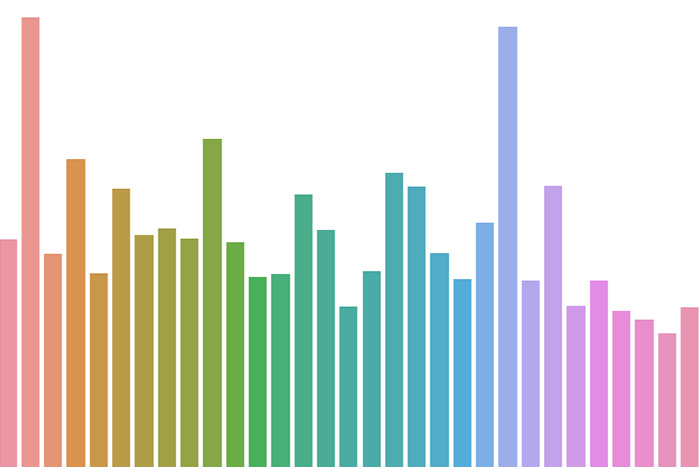# Step-by-Step Data Science Project (End to End Regression Model)

We took “Melbourne housing market dataset from kaggle” and built a model to predict house price. While building the model we found very interesting data patterns such as heteroscedasticity. We tried to solve them by applying transformations on source, target variables. This solved the problems to some extent (still model is under-fitted or high-bias), hence build a more complex Gradient Boosting model, fine-tuned to make model the best.

Below code shows a detailed approach to solve a regression problem. This includes all phases,

1) Business analysis (can we drop “Address”, “CouncilArea”, “Regionname”, “Lattitude”, “Suburb”, “Longtitude” and keep “Postcode” ?)

2) Statistical data analysis (what values should we impute “mean, median, mode” ? – look at descriptive statistics, distribution )

3) Label Encoding, One-hot Encoding (which way to go ? – bi-variate analysis, hypothesis testing)

4) Regression Analysis (what is the relation between “all predictors” and “target” variable)

5)  Analyse root cause (RCA) for under-fitting, try fixing it by applying log transformation to y-variable (heteroscedasticity).

6) Try fitting ensemble model as its a weak learner.

7) Fix over-fitting problem and DONE.

Categories:DataJango - Chatbot